GeeksforGeeks App
Open AppBrowser
Continue

# How to create DataFrame from dictionary in Python-Pandas?

Let’s discuss how to create DataFrame from dictionary in Pandas. There are multiple ways to do this task.

Method 1: Create DataFrame from Dictionary using default Constructor of pandas.Dataframe class.

Code:

 `# import pandas library``import` `pandas as pd`` ` `# dictionary with list object in values``details ``=` `{``    ``'Name'` `: [``'Ankit'``, ``'Aishwarya'``, ``'Shaurya'``, ``'Shivangi'``],``    ``'Age'` `: [``23``, ``21``, ``22``, ``21``],``    ``'University'` `: [``'BHU'``, ``'JNU'``, ``'DU'``, ``'BHU'``],``}`` ` `# creating a Dataframe object ``df ``=` `pd.DataFrame(details)`` ` `df`

Output: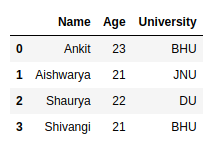Method 2: Create DataFrame from Dictionary with user-defined indexes.

Code:

 `# import pandas library``import` `pandas as pd`` ` `# dictionary with list object in values``details ``=` `{``    ``'Name'` `: [``'Ankit'``, ``'Aishwarya'``, ``'Shaurya'``, ``'Shivangi'``],``    ``'Age'` `: [``23``, ``21``, ``22``, ``21``],``    ``'University'` `: [``'BHU'``, ``'JNU'``, ``'DU'``, ``'BHU'``],``}`` ` `# creating a Dataframe object from dictionary ``# with custom indexing``df ``=` `pd.DataFrame(details, index ``=` `[``'a'``, ``'b'``, ``'c'``, ``'d'``])`` ` `df`

Output: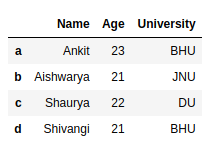Method 3: Create DataFrame from simple dictionary i.e dictionary with key and simple value like integer or string value.

Code:

 `# import pandas library``import` `pandas as pd`` ` `# dictionary``details ``=` `{``    ``'Ankit'` `: ``22``,``    ``'Golu'` `: ``21``,``    ``'hacker'` `: ``23``    ``}`` ` `# creating a Dataframe object from a list ``# of tuples of key, value pair``df ``=` `pd.DataFrame(``list``(details.items()))`` ` `df`

Output: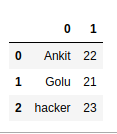Method 4: Create DataFrame from Dictionary with required columns only.

Code:

 `# import pandas library``import` `pandas as pd`` ` `# dictionary with list object in values``details ``=` `{``    ``'Name'` `: [``'Ankit'``, ``'Aishwarya'``, ``'Shaurya'``, ``'Shivangi'``],``    ``'Age'` `: [``23``, ``21``, ``22``, ``21``],``    ``'University'` `: [``'BHU'``, ``'JNU'``, ``'DU'``, ``'BHU'``],``}`` ` `# creating a Dataframe object with skipping ``# one column i.e skipping age column.``df ``=` `pd.DataFrame(details, columns ``=` `[``'Name'``, ``'University'``])`` ` `df`

Output:Method 5: Create DataFrame from Dictionary with different Orientation i.e. Dictionary key is act as indexes in Dataframe.

Code:

 `# import pandas library``import` `pandas as pd`` ` `# dictionary with list object in values``details ``=` `{``    ``'Name'` `: [``'Ankit'``, ``'Aishwarya'``, ``'Shaurya'``, ``'Shivangi'``],``    ``'Age'` `: [``23``, ``21``, ``22``, ``21``],``    ``'University'` `: [``'BHU'``, ``'JNU'``, ``'DU'``, ``'BHU'``],``}`` ` `# creating a Dataframe object in which dictionary``# key is act as index value and column value is``# 0, 1, 2...``df ``=` `pd.DataFrame.from_dict(details, orient ``=` `'index'``)`` ` `df`

Output: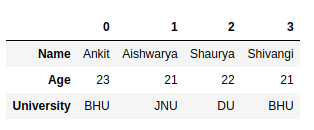Method 6: Create DataFrame from nested Dictionary.

Code:

 `# import pandas library``import` `pandas as pd`` ` `# dictionary with dictionary object``# in values i.e. nested dictionary``details ``=` `{ ``    ``0` `: {``        ``'Name'` `: ``'Ankit'``,``        ``'Age'` `: ``22``,``        ``'University'` `: ``'BHU'``        ``},``    ``1` `: {``        ``'Name'` `: ``'Aishwarya'``,``        ``'Age'` `: ``21``,``        ``'University'` `: ``'JNU'``        ``},``    ``2` `: {``        ``'Name'` `: ``'Shaurya'``,``        ``'Age'` `: ``23``,``        ``'University'` `: ``'DU'``        ``}``}`` ` `# creating a Dataframe object``# from nested dictionary``# in which inside dictionary``# key is act as index value``# and column value is 0, 1, 2...``df ``=` `pd.DataFrame(details)`` ` `# swap the columns with indexes``df ``=` `df.transpose()`` ` `df`

Output: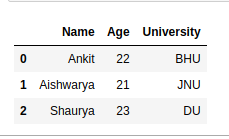My Personal Notes arrow_drop_up